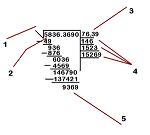# Square root by hand

## Square root by hand step by step

How to calculate a

# SQUARE ROOT

## by handParts of the square root

In the picture we see five essential parts of the square root of the resolution method:

1 – Radical is the symbol that indicates a square root.

2 – Radicand is the number that you get the square root.

3 – Result.

4 – Lines auxiliary help us solve the square root.

5 – Waste, is the end result of the process to solve the square root.

The steps are these:

Step 1: Separate the settled number (in the example, 5836.369) in groups of two digits. The separation is done from the decimal sign (if exists) to the right and to the left. If the side of the decimal (to the right of the point, ie 369) there is an even number of digits, it would add a zero. If the left part of the point, ie, 5836) remain a single digit, don’t worry. In the picture on the right is the number 5836.369 divided into groups of two digits, then the number 9 has added a zero, this separation becomes: 58/36.36/90)

Step 2: Wanted multiplying a number by itself (ie, squared) results match the number that is closest or below the first set of numbers on the left (in the example, 58). The result can not be greater than 58. After finding the number is added to the root. In this case the number would be 7, because 7 × 7 is 49. Another possibility would be 6 × 6, but give 36 (which could be further from 58) and 8 × 8, but give 64 (which would exceed 58)

Step 3: The number obtained (7) is the first result of the square root. In the previous step we wrote in the box on the right. Now multiply it by itself. The result (49) is written below the first set of figures on the left (58), and proceeds to subtract. The result of the subtraction (58-49) is 9. Once the result of the subtraction, lowering the next group of two numbers (36), so that the next digit of the root is now binding previous subtraction result with the new figures decreases (ie, 936 .) To continue the extraction of the square root multiply by 2 the first result (7) and we write just below it, on the next line assistant (pictured, 14 is written just below 7, because 7 × 2 is 14).

Step 4: In this step you have to find a number n that, added to 14, and multiplied by the same number of results in a number less than or equal to 936. That is, it could be 141 × 1, 142 × 2, 143 × 3 … and so up to 149 × 9. Often used trial and error procedure to find that number, although the method can be used to split the first two digits of the residue (93) between the auxiliary line number (14). The first number of non-zero result, even a decimal, is generally we seek. The result is added to the number of the root and the auxiliary line. In this case 93 is divided by 14 6. So the operation sought is 146 × 6 = 876 (add operation in the auxiliary line). The following result is the square root 6. Proceed also to be noted in the filing.

Step 5: The procedure is the same as above. The result of the previous operation (876) is placed below the number subtracted from the previous (936) and subtracted. The result of the subtraction (60) is added to the next set of figures from the radical (in this case, 36). If the next group is after the decimal point decimal point is added to the number of the root. The new number is 6036.

Step 6: We resume the procedure from step 3. The figure of the root (76) is multiplied by two (resulting 152). We look for a number that added to 152 multiplied by that number gives us an approximation to 6036. It would therefore be 1 × 1521, 1522 × 2 1523 × 3, etc.. We can do it by trial and error, or by the process of splitting in this case, the first three digits of the root by the first three digits of the auxiliary line (note that previously were the first two digits), ie 603/152 ( the desired number is 3, since the result is 3.9 and we have said that we should take the figure is the first). The operation to be performed is thus 1523 × 3. The result (4569) is placed under the last bit and proceeds to find the difference (which is 1467). Once the subtraction is low the next set of figures and the process continues. Note that the number of auxiliary line and divide residue is increased.

Step 7: Continue the same process, the root is doubling again (ignoring the decimal point) (763 x 2 = 1526). The multiplication result is added to the third line auxiliary re-split the first four numbers of the residue (1467) between the result of the multiplication (1526), ​​(note that the first four digits are, when previously the first three ), which gives us a score of 0.9 (as we said before, you take the first number that is not zero even decimal, therefore, the amount sought is 9). The nine were added at the root line and the third line auxiliary and multiply 9 by 15 269, giving a score of 137,421, this figure is subtracted from 146 790 and gives a result of 9369.

Source: Wikipedia